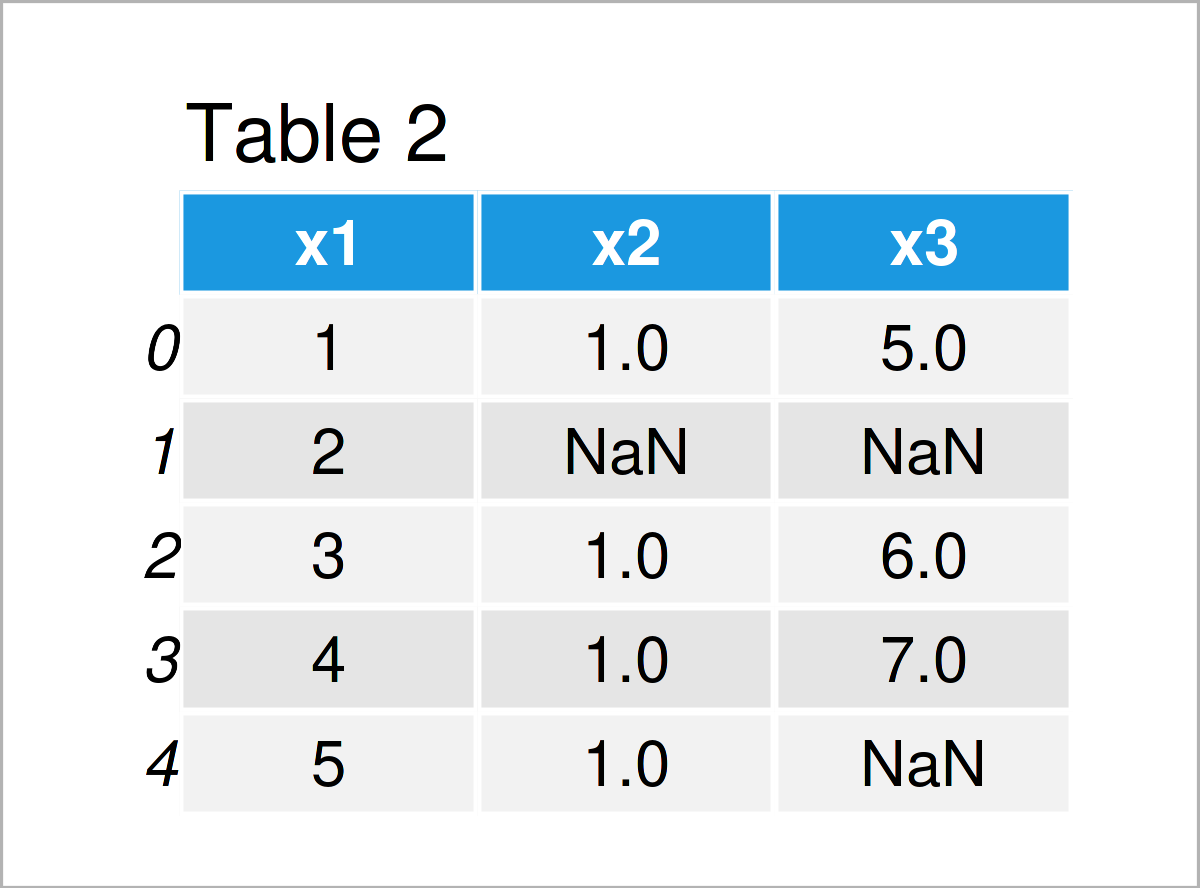# Drop Infinite Values from pandas DataFrame in Python (2 Examples)

In this tutorial you’ll learn how to remove infinite values from a pandas DataFrame in the Python programming language.

Let’s just jump right in!

## Example Data & Software Libraries

First, we have to load the pandas library:

`import pandas as pd                                            # Load pandas library`

`import numpy as np                                             # Import NumPy library in Python`

Furthermore, have a look at the exemplifying data below:

```data = pd.DataFrame({'x1':range(1, 6),                         # Create example DataFrame
'x2':[1, np.inf, 1, 1, 1],
'x3':[5, np.inf, 6, 7, np.inf]})
print(data)                                                    # Print example DataFrame```Table 1 visualizes the output of the Python console and shows that our example data contains five rows and three columns. Some of the cells in our exemplifying DataFrame are infinite (i.e. inf).

## Example 1: Replace inf by NaN in pandas DataFrame

In Example 1, I’ll explain how to exchange the infinite values in a pandas DataFrame by NaN values.

This also needs to be done as first step, in case we want to remove rows with inf values from a data set (more on that in Example 2).

Have a look at the Python code and its output below:

```data_new1 = data.copy()                                        # Create duplicate of data
data_new1.replace([np.inf, - np.inf], np.nan, inplace = True)  # Exchange inf by NaN
print(data_new1)                                               # Print data with NaN```As shown in Table 2, the previous code has created a new pandas DataFrame called data_new1, which contains NaN values instead of inf values.

## Example 2: Remove Rows with NaN Values from pandas DataFrame

This example demonstrates how to drop rows with any NaN values (originally inf values) from a data set.

For this, we can apply the dropna function as shown in the following syntax:

```data_new2 = data_new1.dropna()                                 # Delete rows with NaN
print(data_new2)                                               # Print final data set```After running the previous Python syntax the pandas DataFrame you can see in Table 3 has been created. As you can see, this DataFrame contains fewer lines than the input data, since we have deleted all rows with at least one NaN value.

In case you want to learn more on the removal of NaNs from pandas DataFrames, you can have a look at this tutorial. The tutorials also explains how to remove rows with NaNs in only one specific column.

## Video & Further Resources on this Topic

Do you need further info on the Python code of this article? Then you might have a look at the following video on my YouTube channel. In the video, I’m explaining the content of this post in more detail.

Please accept YouTube cookies to play this video. By accepting you will be accessing content from YouTube, a service provided by an external third party.If you accept this notice, your choice will be saved and the page will refresh.

As an additional resource, you might watch the following video on the YouTube channel of Sebastiaan Mathôt. He’s explaining how to deal with inf and NaN values in the video:

Please accept YouTube cookies to play this video. By accepting you will be accessing content from YouTube, a service provided by an external third party.If you accept this notice, your choice will be saved and the page will refresh.

In addition, you may want to have a look at the other tutorials on my homepage. I have published numerous tutorials already:

Summary: In this post, I have illustrated how to drop infinite values from a pandas DataFrame in the Python programming language. Please let me know in the comments section, in case you have any further questions.

Subscribe to the Statistics Globe Newsletter